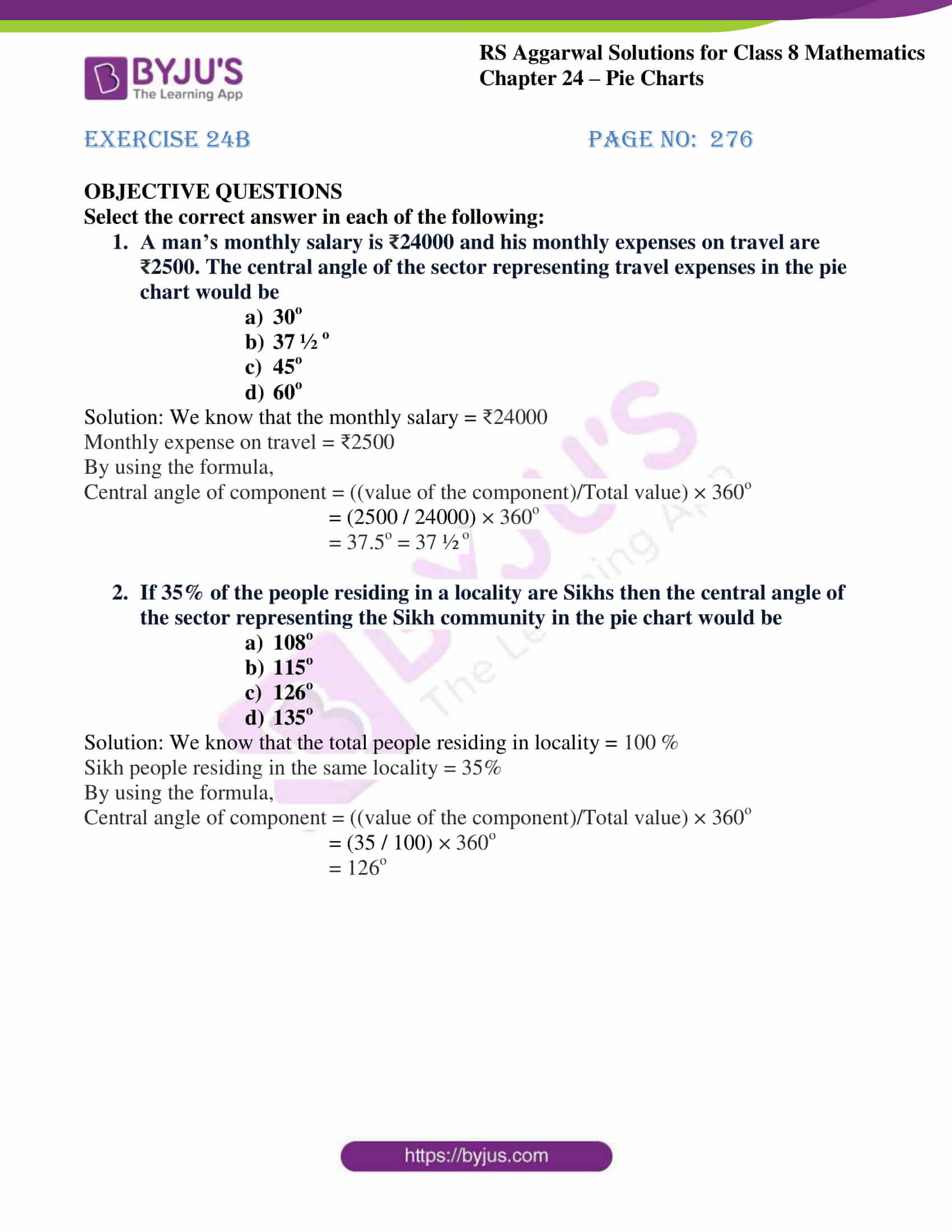# RS Aggarwal Solutions for Class 8 Maths Chapter 24 - Pie Charts Exercise 24B

RS Aggarwal Solutions for Class 8 Maths Chapter 24- Exercise 24B, Pie Charts PDF links are provided below, where students can download effortlessly. Our expert tutors have solved the RS Aggarwal Solutions to help students gain knowledge and secure high marks by practising the solutions. In Exercise 24B of RS Aggarwal Class 8 Maths, we shall brief all the concepts covered in Chapter 24.

## Download PDF of RS Aggarwal Solutions for Class 8 Maths Chapter 24- Pie Charts Exercise 24B### Access Answers to RS Aggarwal Solutions for Class 8 Maths Chapter 24- Pie Charts Exercise 24B

OBJECTIVE QUESTIONS
Select the correct answer in each of the following:
1. A man’s monthly salary is ₹24000 and his monthly expenses on travel are ₹2500. The central angle of the sector representing travel expenses in the pie chart would be

1. 30o
2. 37 ½ o
3. 45o
4. 60o

Solution: We know that the monthly salary = ₹24000
Monthly expense on travel = ₹2500
By using the formula,
Central angle of component = ((value of the component)/Total value) × 360o
= (2500 / 24000) × 360o
= 37.5o = 37 ½ o
2. If 35% of the people residing in a locality are Sikhs then the central angle of the sector representing the Sikh community in the pie chart would be

1. 108o
2. 115o
3. 126o
4. 135o

Solution: We know that the total people residing in locality = 100 %
Sikh people residing in the same locality = 35%
By using the formula,
Central angle of component = ((value of the component)/Total value) × 360o
= (35 / 100) × 360o
= 126o

### Access other Exercise of RS Aggarwal Solutions for Class 8 Maths Chapter 24- Pie Charts

Exercise 24A Solutions 10 Questions

## RS Aggarwal Solutions for Class 8 Maths Chapter 24- Pie Charts Exercise 24B

Exercise 24B of RS Aggarwal Class 8, Pie Charts, mainly consists of objective questions related to the whole Chapter discussed earlier in Chapter 24.

The RS Aggarwal Solutions helps students to understand the fundamental concepts and solve problems with ease. Practising the problems on daily basis will help students to excel in their exams and increase their overall percentage. It also helps students in building time management skills and boost the confidence to appear for the annual exam without any fear.